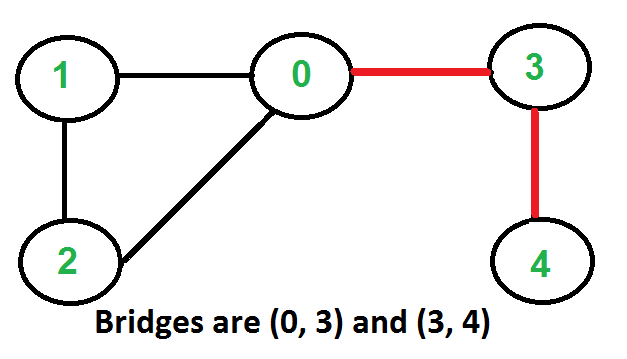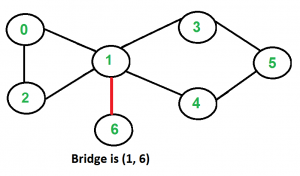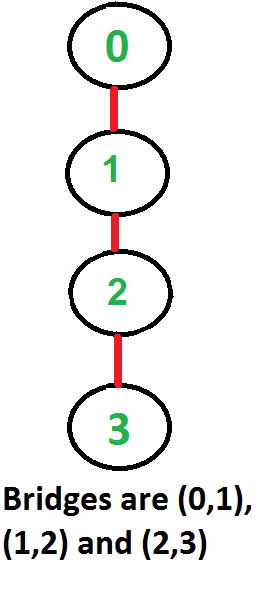Related Articles

# Bridges in a graph

• Difficulty Level : Hard
• Last Updated : 28 Jul, 2021

An edge in an undirected connected graph is a bridge iff removing it disconnects the graph. For a disconnected undirected graph, definition is similar, a bridge is an edge removing which increases number of disconnected components.
Like Articulation Points, bridges represent vulnerabilities in a connected network and are useful for designing reliable networks. For example, in a wired computer network, an articulation point indicates the critical computers and a bridge indicates the critical wires or connections.
Following are some example graphs with bridges highlighted with red color.How to find all bridges in a given graph?
A simple approach is to one by one remove all edges and see if removal of an edge causes disconnected graph. Following are steps of simple approach for connected graph.
1) For every edge (u, v), do following
…..a) Remove (u, v) from graph
..…b) See if the graph remains connected (We can either use BFS or DFS)
…..c) Add (u, v) back to the graph.
Time complexity of above method is O(E*(V+E)) for a graph represented using adjacency list. Can we do better?
A O(V+E) algorithm to find all Bridges
The idea is similar to O(V+E) algorithm for Articulation Points. We do DFS traversal of the given graph. In DFS tree an edge (u, v) (u is parent of v in DFS tree) is bridge if there does not exist any other alternative to reach u or an ancestor of u from subtree rooted with v. As discussed in the previous post, the value low[v] indicates earliest visited vertex reachable from subtree rooted with v. The condition for an edge (u, v) to be a bridge is, “low[v] > disc[u]”
Following are C++ and Java implementations of above approach.

## C++

 `// A C++ program to find bridges in a given undirected graph``#include``#include ``#define NIL -1``using` `namespace` `std;` `// A class that represents an undirected graph``class` `Graph``{``    ``int` `V;    ``// No. of vertices``    ``list<``int``> *adj;    ``// A dynamic array of adjacency lists``    ``void` `bridgeUtil(``int` `v, ``bool` `visited[], ``int` `disc[], ``int` `low[],``                    ``int` `parent[]);``public``:``    ``Graph(``int` `V);   ``// Constructor``    ``void` `addEdge(``int` `v, ``int` `w);   ``// to add an edge to graph``    ``void` `bridge();    ``// prints all bridges``};` `Graph::Graph(``int` `V)``{``    ``this``->V = V;``    ``adj = ``new` `list<``int``>[V];``}` `void` `Graph::addEdge(``int` `v, ``int` `w)``{``    ``adj[v].push_back(w);``    ``adj[w].push_back(v);  ``// Note: the graph is undirected``}` `// A recursive function that finds and prints bridges using``// DFS traversal``// u --> The vertex to be visited next``// visited[] --> keeps tract of visited vertices``// disc[] --> Stores discovery times of visited vertices``// parent[] --> Stores parent vertices in DFS tree``void` `Graph::bridgeUtil(``int` `u, ``bool` `visited[], ``int` `disc[],``                                  ``int` `low[], ``int` `parent[])``{``    ``// A static variable is used for simplicity, we can``    ``// avoid use of static variable by passing a pointer.``    ``static` `int` `time` `= 0;` `    ``// Mark the current node as visited``    ``visited[u] = ``true``;` `    ``// Initialize discovery time and low value``    ``disc[u] = low[u] = ++``time``;` `    ``// Go through all vertices aadjacent to this``    ``list<``int``>::iterator i;``    ``for` `(i = adj[u].begin(); i != adj[u].end(); ++i)``    ``{``        ``int` `v = *i;  ``// v is current adjacent of u` `        ``// If v is not visited yet, then recur for it``        ``if` `(!visited[v])``        ``{``            ``parent[v] = u;``            ``bridgeUtil(v, visited, disc, low, parent);` `            ``// Check if the subtree rooted with v has a``            ``// connection to one of the ancestors of u``            ``low[u]  = min(low[u], low[v]);` `            ``// If the lowest vertex reachable from subtree``            ``// under v is  below u in DFS tree, then u-v``            ``// is a bridge``            ``if` `(low[v] > disc[u])``              ``cout << u <<``" "` `<< v << endl;``        ``}` `        ``// Update low value of u for parent function calls.``        ``else` `if` `(v != parent[u])``            ``low[u]  = min(low[u], disc[v]);``    ``}``}` `// DFS based function to find all bridges. It uses recursive``// function bridgeUtil()``void` `Graph::bridge()``{``    ``// Mark all the vertices as not visited``    ``bool` `*visited = ``new` `bool``[V];``    ``int` `*disc = ``new` `int``[V];``    ``int` `*low = ``new` `int``[V];``    ``int` `*parent = ``new` `int``[V];` `    ``// Initialize parent and visited arrays``    ``for` `(``int` `i = 0; i < V; i++)``    ``{``        ``parent[i] = NIL;``        ``visited[i] = ``false``;``    ``}` `    ``// Call the recursive helper function to find Bridges``    ``// in DFS tree rooted with vertex 'i'``    ``for` `(``int` `i = 0; i < V; i++)``        ``if` `(visited[i] == ``false``)``            ``bridgeUtil(i, visited, disc, low, parent);``}` `// Driver program to test above function``int` `main()``{``    ``// Create graphs given in above diagrams``    ``cout << ``"\nBridges in first graph \n"``;``    ``Graph g1(5);``    ``g1.addEdge(1, 0);``    ``g1.addEdge(0, 2);``    ``g1.addEdge(2, 1);``    ``g1.addEdge(0, 3);``    ``g1.addEdge(3, 4);``    ``g1.bridge();` `    ``cout << ``"\nBridges in second graph \n"``;``    ``Graph g2(4);``    ``g2.addEdge(0, 1);``    ``g2.addEdge(1, 2);``    ``g2.addEdge(2, 3);``    ``g2.bridge();` `    ``cout << ``"\nBridges in third graph \n"``;``    ``Graph g3(7);``    ``g3.addEdge(0, 1);``    ``g3.addEdge(1, 2);``    ``g3.addEdge(2, 0);``    ``g3.addEdge(1, 3);``    ``g3.addEdge(1, 4);``    ``g3.addEdge(1, 6);``    ``g3.addEdge(3, 5);``    ``g3.addEdge(4, 5);``    ``g3.bridge();` `    ``return` `0;``}`

## Java

 `// A Java program to find bridges in a given undirected graph``import` `java.io.*;``import` `java.util.*;``import` `java.util.LinkedList;` `// This class represents a undirected graph using adjacency list``// representation``class` `Graph``{``    ``private` `int` `V;   ``// No. of vertices` `    ``// Array  of lists for Adjacency List Representation``    ``private` `LinkedList adj[];``    ``int` `time = ``0``;``    ``static` `final` `int` `NIL = -``1``;` `    ``// Constructor``    ``Graph(``int` `v)``    ``{``        ``V = v;``        ``adj = ``new` `LinkedList[v];``        ``for` `(``int` `i=``0``; i The vertex to be visited next``    ``// visited[] --> keeps tract of visited vertices``    ``// disc[] --> Stores discovery times of visited vertices``    ``// parent[] --> Stores parent vertices in DFS tree``    ``void` `bridgeUtil(``int` `u, ``boolean` `visited[], ``int` `disc[],``                    ``int` `low[], ``int` `parent[])``    ``{` `        ``// Mark the current node as visited``        ``visited[u] = ``true``;` `        ``// Initialize discovery time and low value``        ``disc[u] = low[u] = ++time;` `        ``// Go through all vertices aadjacent to this``        ``Iterator i = adj[u].iterator();``        ``while` `(i.hasNext())``        ``{``            ``int` `v = i.next();  ``// v is current adjacent of u` `            ``// If v is not visited yet, then make it a child``            ``// of u in DFS tree and recur for it.``            ``// If v is not visited yet, then recur for it``            ``if` `(!visited[v])``            ``{``                ``parent[v] = u;``                ``bridgeUtil(v, visited, disc, low, parent);` `                ``// Check if the subtree rooted with v has a``                ``// connection to one of the ancestors of u``                ``low[u]  = Math.min(low[u], low[v]);` `                ``// If the lowest vertex reachable from subtree``                ``// under v is below u in DFS tree, then u-v is``                ``// a bridge``                ``if` `(low[v] > disc[u])``                    ``System.out.println(u+``" "``+v);``            ``}` `            ``// Update low value of u for parent function calls.``            ``else` `if` `(v != parent[u])``                ``low[u]  = Math.min(low[u], disc[v]);``        ``}``    ``}`  `    ``// DFS based function to find all bridges. It uses recursive``    ``// function bridgeUtil()``    ``void` `bridge()``    ``{``        ``// Mark all the vertices as not visited``        ``boolean` `visited[] = ``new` `boolean``[V];``        ``int` `disc[] = ``new` `int``[V];``        ``int` `low[] = ``new` `int``[V];``        ``int` `parent[] = ``new` `int``[V];`  `        ``// Initialize parent and visited, and ap(articulation point)``        ``// arrays``        ``for` `(``int` `i = ``0``; i < V; i++)``        ``{``            ``parent[i] = NIL;``            ``visited[i] = ``false``;``        ``}` `        ``// Call the recursive helper function to find Bridges``        ``// in DFS tree rooted with vertex 'i'``        ``for` `(``int` `i = ``0``; i < V; i++)``            ``if` `(visited[i] == ``false``)``                ``bridgeUtil(i, visited, disc, low, parent);``    ``}` `    ``public` `static` `void` `main(String args[])``    ``{``        ``// Create graphs given in above diagrams``        ``System.out.println(``"Bridges in first graph "``);``        ``Graph g1 = ``new` `Graph(``5``);``        ``g1.addEdge(``1``, ``0``);``        ``g1.addEdge(``0``, ``2``);``        ``g1.addEdge(``2``, ``1``);``        ``g1.addEdge(``0``, ``3``);``        ``g1.addEdge(``3``, ``4``);``        ``g1.bridge();``        ``System.out.println();` `        ``System.out.println(``"Bridges in Second graph"``);``        ``Graph g2 = ``new` `Graph(``4``);``        ``g2.addEdge(``0``, ``1``);``        ``g2.addEdge(``1``, ``2``);``        ``g2.addEdge(``2``, ``3``);``        ``g2.bridge();``        ``System.out.println();` `        ``System.out.println(``"Bridges in Third graph "``);``        ``Graph g3 = ``new` `Graph(``7``);``        ``g3.addEdge(``0``, ``1``);``        ``g3.addEdge(``1``, ``2``);``        ``g3.addEdge(``2``, ``0``);``        ``g3.addEdge(``1``, ``3``);``        ``g3.addEdge(``1``, ``4``);``        ``g3.addEdge(``1``, ``6``);``        ``g3.addEdge(``3``, ``5``);``        ``g3.addEdge(``4``, ``5``);``        ``g3.bridge();``    ``}``}``// This code is contributed by Aakash Hasija`

## Python

 `# Python program to find bridges in a given undirected graph``#Complexity : O(V+E)`` ` `from` `collections ``import` `defaultdict`` ` `#This class represents an undirected graph using adjacency list representation``class` `Graph:`` ` `    ``def` `__init__(``self``,vertices):``        ``self``.V``=` `vertices ``#No. of vertices``        ``self``.graph ``=` `defaultdict(``list``) ``# default dictionary to store graph``        ``self``.Time ``=` `0`` ` `    ``# function to add an edge to graph``    ``def` `addEdge(``self``,u,v):``        ``self``.graph[u].append(v)``        ``self``.graph[v].append(u)`` ` `    ``'''A recursive function that finds and prints bridges``    ``using DFS traversal``    ``u --> The vertex to be visited next``    ``visited[] --> keeps tract of visited vertices``    ``disc[] --> Stores discovery times of visited vertices``    ``parent[] --> Stores parent vertices in DFS tree'''``    ``def` `bridgeUtil(``self``,u, visited, parent, low, disc):` `        ``# Mark the current node as visited and print it``        ``visited[u]``=` `True` `        ``# Initialize discovery time and low value``        ``disc[u] ``=` `self``.Time``        ``low[u] ``=` `self``.Time``        ``self``.Time ``+``=` `1` `        ``#Recur for all the vertices adjacent to this vertex``        ``for` `v ``in` `self``.graph[u]:``            ``# If v is not visited yet, then make it a child of u``            ``# in DFS tree and recur for it``            ``if` `visited[v] ``=``=` `False` `:``                ``parent[v] ``=` `u``                ``self``.bridgeUtil(v, visited, parent, low, disc)` `                ``# Check if the subtree rooted with v has a connection to``                ``# one of the ancestors of u``                ``low[u] ``=` `min``(low[u], low[v])`  `                ``''' If the lowest vertex reachable from subtree``                ``under v is below u in DFS tree, then u-v is``                ``a bridge'''``                ``if` `low[v] > disc[u]:``                    ``print` `(``"%d %d"` `%``(u,v))``    ` `                    ` `            ``elif` `v !``=` `parent[u]: ``# Update low value of u for parent function calls.``                ``low[u] ``=` `min``(low[u], disc[v])`  `    ``# DFS based function to find all bridges. It uses recursive``    ``# function bridgeUtil()``    ``def` `bridge(``self``):`` ` `        ``# Mark all the vertices as not visited and Initialize parent and visited,``        ``# and ap(articulation point) arrays``        ``visited ``=` `[``False``] ``*` `(``self``.V)``        ``disc ``=` `[``float``(``"Inf"``)] ``*` `(``self``.V)``        ``low ``=` `[``float``(``"Inf"``)] ``*` `(``self``.V)``        ``parent ``=` `[``-``1``] ``*` `(``self``.V)` `        ``# Call the recursive helper function to find bridges``        ``# in DFS tree rooted with vertex 'i'``        ``for` `i ``in` `range``(``self``.V):``            ``if` `visited[i] ``=``=` `False``:``                ``self``.bridgeUtil(i, visited, parent, low, disc)``        ` ` ` `# Create a graph given in the above diagram``g1 ``=` `Graph(``5``)``g1.addEdge(``1``, ``0``)``g1.addEdge(``0``, ``2``)``g1.addEdge(``2``, ``1``)``g1.addEdge(``0``, ``3``)``g1.addEdge(``3``, ``4``)` ` ` `print` `"Bridges in first graph "``g1.bridge()` `g2 ``=` `Graph(``4``)``g2.addEdge(``0``, ``1``)``g2.addEdge(``1``, ``2``)``g2.addEdge(``2``, ``3``)``print` `"\nBridges in second graph "``g2.bridge()` ` ` `g3 ``=` `Graph (``7``)``g3.addEdge(``0``, ``1``)``g3.addEdge(``1``, ``2``)``g3.addEdge(``2``, ``0``)``g3.addEdge(``1``, ``3``)``g3.addEdge(``1``, ``4``)``g3.addEdge(``1``, ``6``)``g3.addEdge(``3``, ``5``)``g3.addEdge(``4``, ``5``)``print` `"\nBridges in third graph "``g3.bridge()`  `#This code is contributed by Neelam Yadav`

## C#

 `// A C# program to find bridges``// in a given undirected graph``using` `System;``using` `System.Collections.Generic;` `// This class represents a undirected graph ``// using adjacency list representation``public` `class` `Graph``{``    ``private` `int` `V; ``// No. of vertices` `    ``// Array of lists for Adjacency List Representation``    ``private` `List<``int``> []adj;``    ``int` `time = 0;``    ``static` `readonly` `int` `NIL = -1;` `    ``// Constructor``    ``Graph(``int` `v)``    ``{``        ``V = v;``        ``adj = ``new` `List<``int``>[v];``        ``for` `(``int` `i = 0; i < v; ++i)``            ``adj[i] = ``new` `List<``int``>();``    ``}` `    ``// Function to add an edge into the graph``    ``void` `addEdge(``int` `v, ``int` `w)``    ``{``        ``adj[v].Add(w); ``// Add w to v's list.``        ``adj[w].Add(v); ``//Add v to w's list``    ``}` `    ``// A recursive function that finds and prints bridges``    ``// using DFS traversal``    ``// u --> The vertex to be visited next``    ``// visited[] --> keeps tract of visited vertices``    ``// disc[] --> Stores discovery times of visited vertices``    ``// parent[] --> Stores parent vertices in DFS tree``    ``void` `bridgeUtil(``int` `u, ``bool` `[]visited, ``int` `[]disc,``                    ``int` `[]low, ``int` `[]parent)``    ``{` `        ``// Mark the current node as visited``        ``visited[u] = ``true``;` `        ``// Initialize discovery time and low value``        ``disc[u] = low[u] = ++time;` `        ``// Go through all vertices aadjacent to this``        ``foreach``(``int` `i ``in` `adj[u])``        ``{``            ``int` `v = i; ``// v is current adjacent of u` `            ``// If v is not visited yet, then make it a child``            ``// of u in DFS tree and recur for it.``            ``// If v is not visited yet, then recur for it``            ``if` `(!visited[v])``            ``{``                ``parent[v] = u;``                ``bridgeUtil(v, visited, disc, low, parent);` `                ``// Check if the subtree rooted with v has a``                ``// connection to one of the ancestors of u``                ``low[u] = Math.Min(low[u], low[v]);` `                ``// If the lowest vertex reachable from subtree``                ``// under v is below u in DFS tree, then u-v is``                ``// a bridge``                ``if` `(low[v] > disc[u])``                    ``Console.WriteLine(u + ``" "` `+ v);``            ``}` `            ``// Update low value of u for parent function calls.``            ``else` `if` `(v != parent[u])``                ``low[u] = Math.Min(low[u], disc[v]);``        ``}``    ``}`  `    ``// DFS based function to find all bridges. It uses recursive``    ``// function bridgeUtil()``    ``void` `bridge()``    ``{``        ``// Mark all the vertices as not visited``        ``bool` `[]visited = ``new` `bool``[V];``        ``int` `[]disc = ``new` `int``[V];``        ``int` `[]low = ``new` `int``[V];``        ``int` `[]parent = ``new` `int``[V];`  `        ``// Initialize parent and visited, ``        ``// and ap(articulation point) arrays``        ``for` `(``int` `i = 0; i < V; i++)``        ``{``            ``parent[i] = NIL;``            ``visited[i] = ``false``;``        ``}` `        ``// Call the recursive helper function to find Bridges``        ``// in DFS tree rooted with vertex 'i'``        ``for` `(``int` `i = 0; i < V; i++)``            ``if` `(visited[i] == ``false``)``                ``bridgeUtil(i, visited, disc, low, parent);``    ``}` `    ``// Driver code``    ``public` `static` `void` `Main(String []args)``    ``{``        ``// Create graphs given in above diagrams``        ``Console.WriteLine(``"Bridges in first graph "``);``        ``Graph g1 = ``new` `Graph(5);``        ``g1.addEdge(1, 0);``        ``g1.addEdge(0, 2);``        ``g1.addEdge(2, 1);``        ``g1.addEdge(0, 3);``        ``g1.addEdge(3, 4);``        ``g1.bridge();``        ``Console.WriteLine();` `        ``Console.WriteLine(``"Bridges in Second graph"``);``        ``Graph g2 = ``new` `Graph(4);``        ``g2.addEdge(0, 1);``        ``g2.addEdge(1, 2);``        ``g2.addEdge(2, 3);``        ``g2.bridge();``        ``Console.WriteLine();` `        ``Console.WriteLine(``"Bridges in Third graph "``);``        ``Graph g3 = ``new` `Graph(7);``        ``g3.addEdge(0, 1);``        ``g3.addEdge(1, 2);``        ``g3.addEdge(2, 0);``        ``g3.addEdge(1, 3);``        ``g3.addEdge(1, 4);``        ``g3.addEdge(1, 6);``        ``g3.addEdge(3, 5);``        ``g3.addEdge(4, 5);``        ``g3.bridge();``    ``}``}` `// This code is contributed by Rajput-Ji`

## Javascript

 ``

Output:

```Bridges in first graph
3 4
0 3

Bridges in second graph
2 3
1 2
0 1

Bridges in third graph
1 6```

Time Complexity: The above function is simple DFS with additional arrays. So time complexity is same as DFS which is O(V+E) for adjacency list representation of graph.

References:
https://www.cs.washington.edu/education/courses/421/04su/slides/artic.pdf
http://www.slideshare.net/TraianRebedea/algorithm-design-and-complexity-course-8
http://faculty.simpson.edu/lydia.sinapova/www/cmsc250/LN250_Weiss/L25-Connectivity.htm